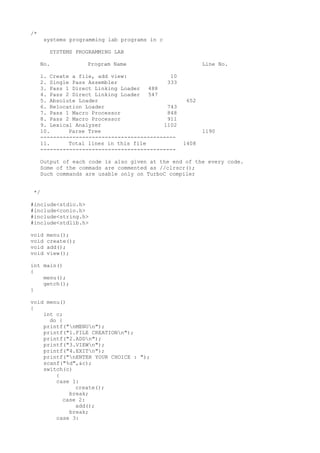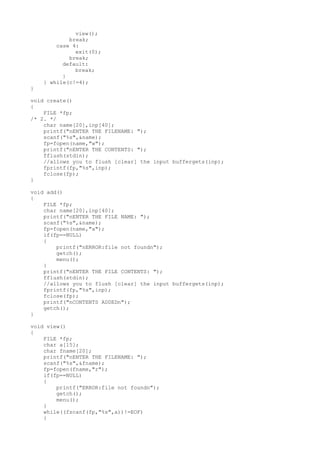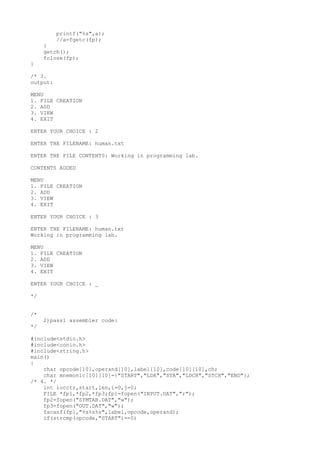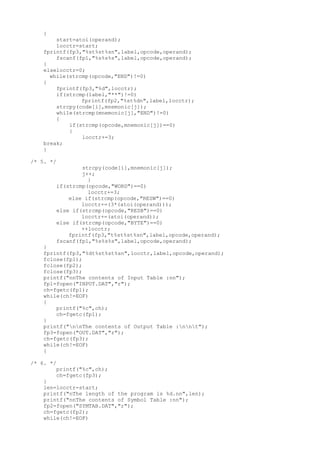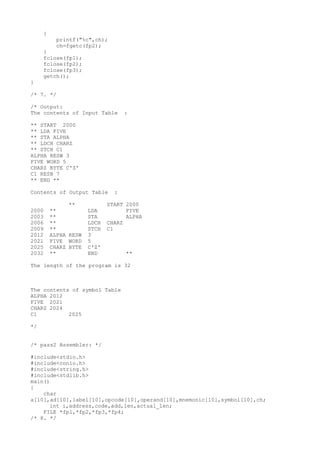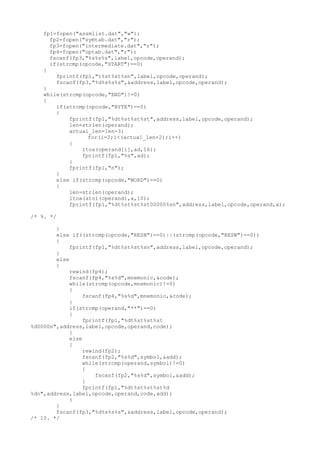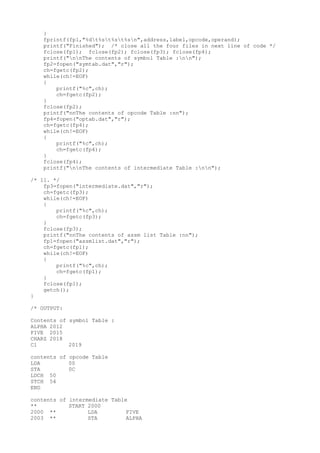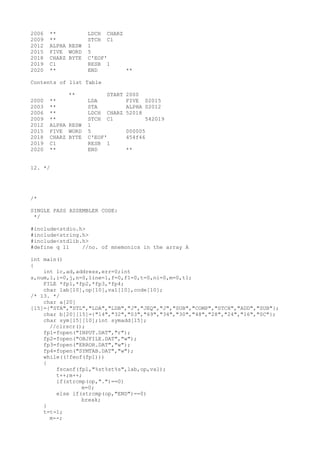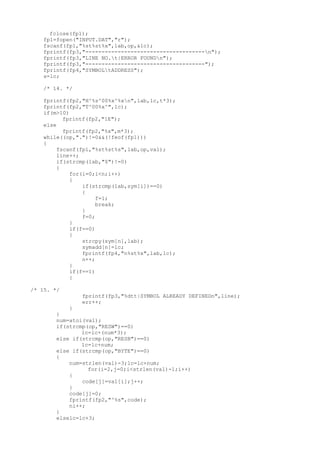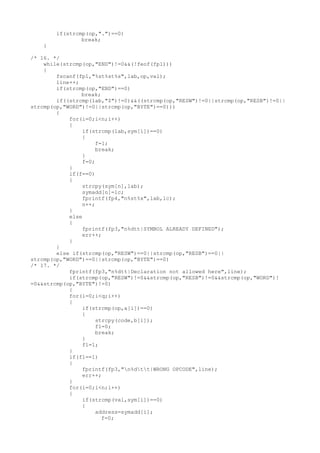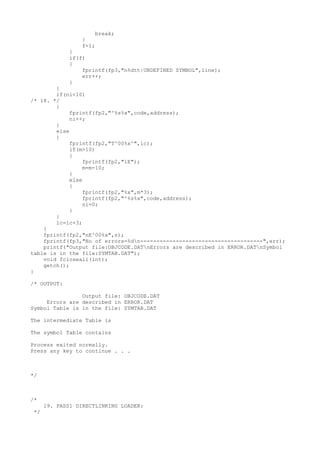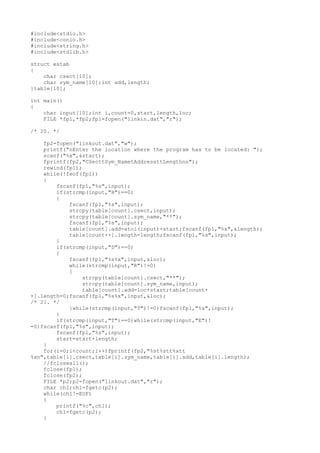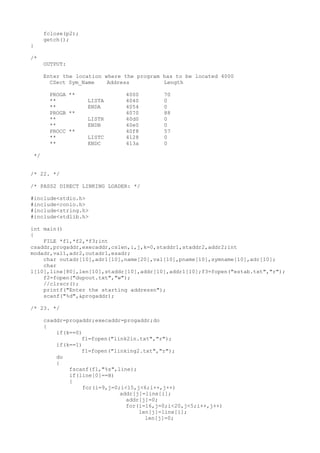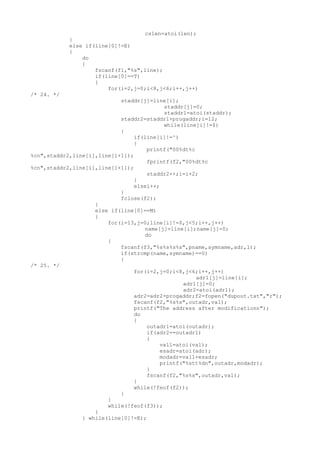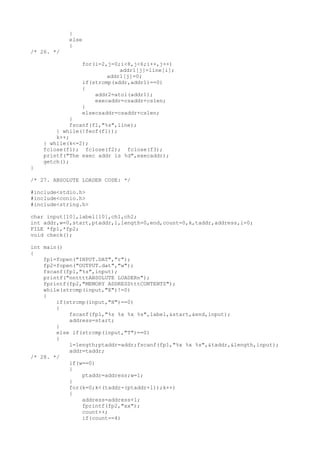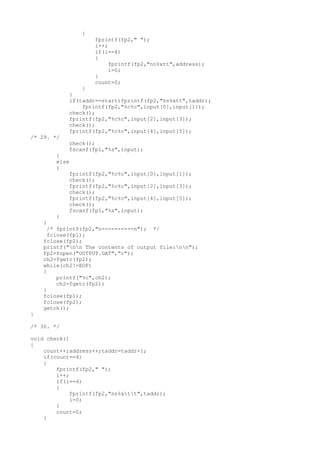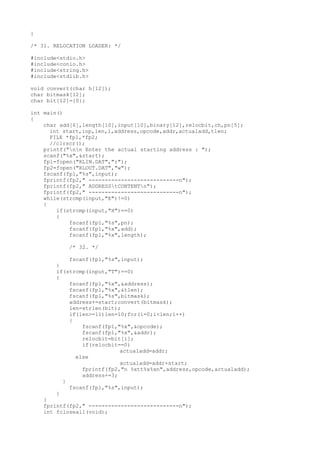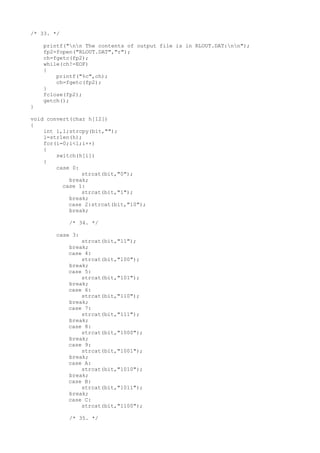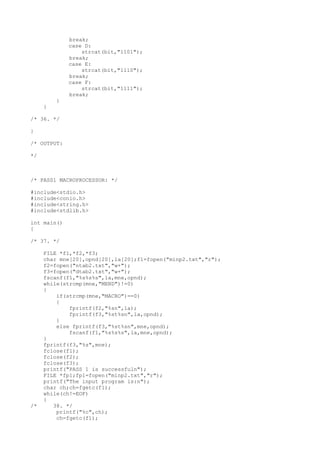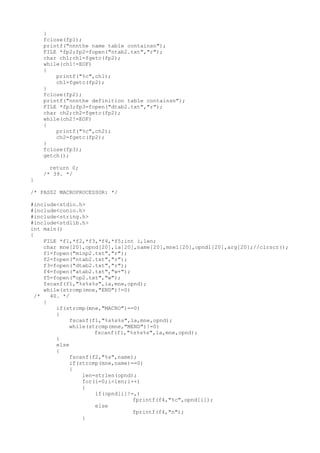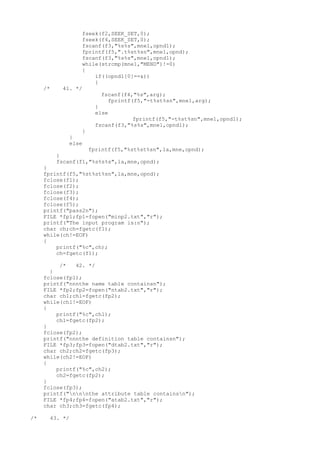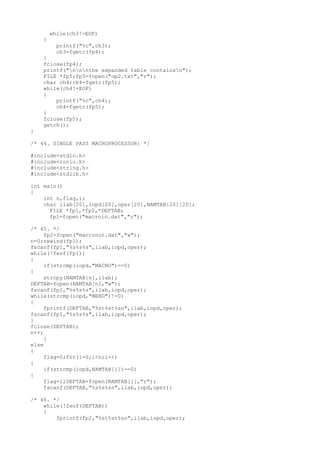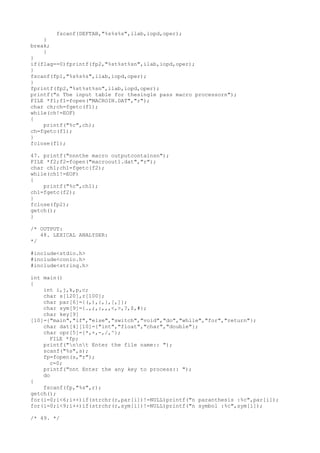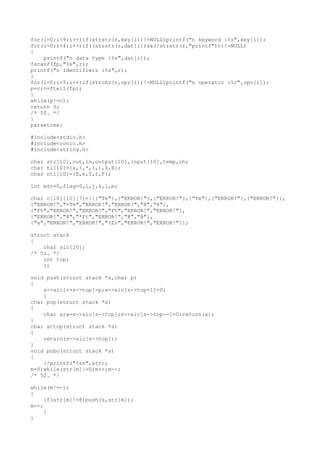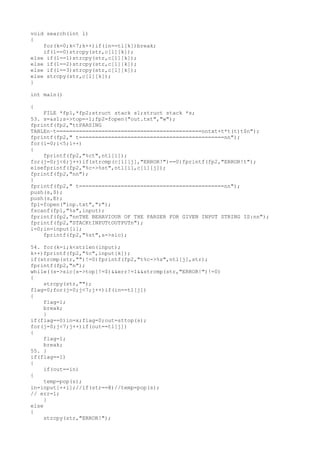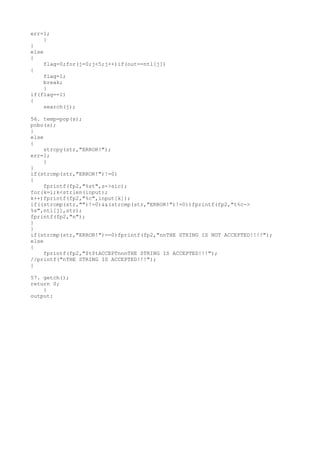Upcoming SlideShare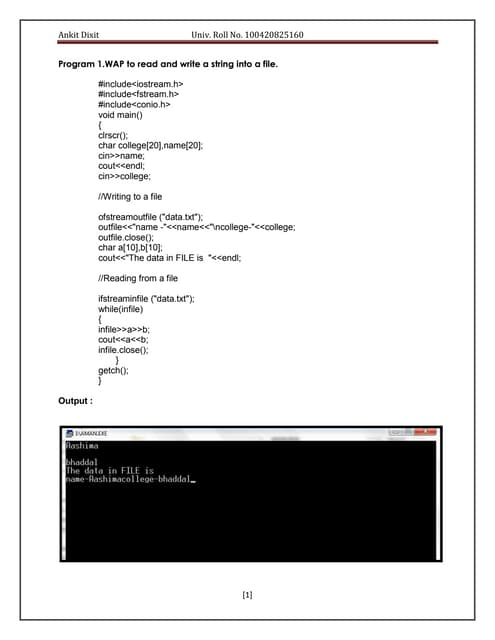System programmin practical file
1 of 26

### System programs in C language.

2. view(); break; case 4: exit(0); break; default: break; } } while(c!=4); } void create() { FILE *fp; /* 2. */ char name,inp; printf("nENTER THE FILENAME: "); scanf("%s",&name); fp=fopen(name,"w"); printf("nENTER THE CONTENTS: "); fflush(stdin); //allows you to flush [clear] the input buffergets(inp); fprintf(fp,"%s",inp); fclose(fp); } void add() { FILE *fp; char name,inp; printf("nENTER THE FILE NAME: "); scanf("%s",&name); fp=fopen(name,"a"); if(fp==NULL) { printf("nERROR:file not foundn"); getch(); menu(); } printf("nENTER THE FILE CONTENTS: "); fflush(stdin); //allows you to flush [clear] the input buffergets(inp); fprintf(fp,"%s",inp); fclose(fp); printf("nCONTENTS ADDEDn"); getch(); } void view() { FILE *fp; char a; char fname; printf("nENTER THE FILENAME: "); scanf("%s",&fname); fp=fopen(fname,"r"); if(fp==NULL) { printf("ERROR:file not foundn"); getch(); menu(); } while((fscanf(fp,"%s",a))!=EOF) {
3. printf("%s",a); //a=fgetc(fp); } getch(); fclose(fp); } /* 3. output: MENU 1. FILE CREATION 2. ADD 3. VIEW 4. EXIT ENTER YOUR CHOICE : 2 ENTER THE FILENAME: human.txt ENTER THE FILE CONTENTS: Working in programming lab. CONTENTS ADDED MENU 1. FILE CREATION 2. ADD 3. VIEW 4. EXIT ENTER YOUR CHOICE : 3 ENTER THE FILENAME: human.txt Working in programming lab. MENU 1. FILE CREATION 2. ADD 3. VIEW 4. EXIT ENTER YOUR CHOICE : _ */ /* 2)pass1 assembler code: */ #include<stdio.h> #include<conio.h> #include<string.h> main() { char opcode,operand,label,code,ch; char mnemonic={"START","LDA","STA","LDCH","STCH","END"}; /* 4. */ int locctr,start,len,i=0,j=0; FILE *fp1,*fp2,*fp3;fp1=fopen("INPUT.DAT","r"); fp2=fopen("SYMTAB.DAT","w"); fp3=fopen("OUT.DAT","w"); fscanf(fp1,"%s%s%s",label,opcode,operand); if(strcmp(opcode,"START")==0)
4. { start=atoi(operand); locctr=start; fprintf(fp3,"%st%st%sn",label,opcode,operand); fscanf(fp1,"%s%s%s",label,opcode,operand); } elselocctr=0; while(strcmp(opcode,"END")!=0) { fprintf(fp3,"%d",locctr); if(strcmp(label,"**")!=0) fprintf(fp2,"%st%dn",label,locctr); strcpy(code[i],mnemonic[j]); while(strcmp(mnemonic[j],"END")!=0) { if(strcmp(opcode,mnemonic[j])==0) { locctr+=3; break; } /* 5. */ strcpy(code[i],mnemonic[j]); j++; } if(strcmp(opcode,"WORD")==0) locctr+=3; else if(strcmp(opcode,"RESW")==0) locctr+=(3*(atoi(operand))); else if(strcmp(opcode,"RESB")==0) locctr+=(atoi(operand)); else if(strcmp(opcode,"BYTE")==0) ++locctr; fprintf(fp3,"t%st%st%sn",label,opcode,operand); fscanf(fp1,"%s%s%s",label,opcode,operand); } fprintf(fp3,"%dt%st%st%sn",locctr,label,opcode,operand); fclose(fp1); fclose(fp2); fclose(fp3); printf("nnThe contents of Input Table :nn"); fp1=fopen("INPUT.DAT","r"); ch=fgetc(fp1); while(ch!=EOF) { printf("%c",ch); ch=fgetc(fp1); } printf("nnThe contents of Output Table :nnt"); fp3=fopen("OUT.DAT","r"); ch=fgetc(fp3); while(ch!=EOF) { /* 6. */ printf("%c",ch); ch=fgetc(fp3); } len=locctr-start; printf("nThe length of the program is %d.nn",len); printf("nnThe contents of Symbol Table :nn"); fp2=fopen("SYMTAB.DAT","r"); ch=fgetc(fp2); while(ch!=EOF)
5. { printf("%c",ch); ch=fgetc(fp2); } fclose(fp1); fclose(fp2); fclose(fp3); getch(); } /* 7. */ /* Output: The contents of Input Table : ** START 2000 ** LDA FIVE ** STA ALPHA ** LDCH CHARZ ** STCH C1 ALPHA RESW 3 FIVE WORD 5 CHARZ BYTE C'Z' C1 RESB 7 ** END ** Contents of Output Table : ** START 2000 2000 ** LDA FIVE 2003 ** STA ALPHA 2006 ** LDCH CHARZ 2009 ** STCH C1 2012 ALPHA RESW 3 2021 FIVE WORD 5 2025 CHARZ BYTE C'Z' 2032 ** END ** The length of the program is 32 The contents of symbol Table ALPHA 2012 FIVE 2021 CHARZ 2024 C1 2025 */ /* pass2 Assembler: */ #include<stdio.h> #include<conio.h> #include<string.h> #include<stdlib.h> main() { char a,ad,label,opcode,operand,mnemonic,symbol,ch; int i,address,code,add,len,actual_len; FILE *fp1,*fp2,*fp3,*fp4; /* 8. */
7. } fprintf(fp1,"%dt%st%st%sn",address,label,opcode,operand); printf("Finished"); /* close all the four files in next line of code */ fclose(fp1); fclose(fp2); fclose(fp3); fclose(fp4); printf("nnThe contents of symbol Table :nn"); fp2=fopen("symtab.dat","r"); ch=fgetc(fp2); while(ch!=EOF) { printf("%c",ch); ch=fgetc(fp2); } fclose(fp2); printf("nnThe contents of opcode Table :nn"); fp4=fopen("optab.dat","r"); ch=fgetc(fp4); while(ch!=EOF) { printf("%c",ch); ch=fgetc(fp4); } fclose(fp4); printf("nnThe contents of intermediate Table :nn"); /* 11. */ fp3=fopen("intermediate.dat","r"); ch=fgetc(fp3); while(ch!=EOF) { printf("%c",ch); ch=fgetc(fp3); } fclose(fp3); printf("nnThe contents of assm list Table :nn"); fp1=fopen("assmlist.dat","r"); ch=fgetc(fp1); while(ch!=EOF) { printf("%c",ch); ch=fgetc(fp1); } fclose(fp1); getch(); } /* OUTPUT: Contents of symbol Table : ALPHA 2012 FIVE 2015 CHARZ 2018 C1 2019 contents of opcode Table LDA 00 STA 0C LDCH 50 STCH 54 END contents of intermediate Table ** START 2000 2000 ** LDA FIVE 2003 ** STA ALPHA
8. 2006 ** LDCH CHARZ 2009 ** STCH C1 2012 ALPHA RESW 1 2015 FIVE WORD 5 2018 CHARZ BYTE C'EOF' 2019 C1 RESB 1 2020 ** END ** Contents of list Table ** START 2000 2000 ** LDA FIVE 02015 2003 ** STA ALPHA 02012 2006 ** LDCH CHARZ 52018 2009 ** STCH C1 542019 2012 ALPHA RESW 1 2015 FIVE WORD 5 000005 2018 CHARZ BYTE C'EOF' 454f46 2019 C1 RESB 1 2020 ** END ** 12. */ /* SINGLE PASS ASSEMBLER CODE: */ #include<stdio.h> #include<string.h> #include<stdlib.h> #define q 11 //no. of mnemonics in the array A int main() { int lc,ad,address,err=0;int s,num,l,i=0,j,n=0,line=1,f=0,f1=0,t=0,ni=0,m=0,t1; FILE *fp1,*fp2,*fp3,*fp4; char lab,op,val,code; /* 13. */ char a ={"STA","STL","LDA","LDB","J","JEQ","J","SUB","COMP","STCH","ADD","SUB"}; char b={"14","32","03","69","34","30","48","28","24","16","0C"}; char sym;int symadd; //clrscr(); fp1=fopen("INPUT.DAT","r"); fp2=fopen("OBJFILE.DAT","w"); fp3=fopen("ERROR.DAT","w"); fp4=fopen("SYMTAB.DAT","w"); while((!feof(fp1))) { fscanf(fp1,"%st%st%s",lab,op,val); t++;m++; if(strcmp(op,".")==0) m=0; else if(strcmp(op,"END")==0) break; } t=t-1; m--;
9. fclose(fp1); fp1=fopen("INPUT.DAT","r"); fscanf(fp1,"%st%st%x",lab,op,&lc); fprintf(fp3,"-------------------------------------n"); fprintf(fp3,"LINE NO.t|ERROR FOUNDn"); fprintf(fp3,"-------------------------------------"); fprintf(fp4,"SYMBOLtADDRESS"); s=lc; /* 14. */ fprintf(fp2,"H^%s^00%x^%xn",lab,lc,t*3); fprintf(fp2,"T^00%x^",lc); if(m>10) fprintf(fp2,"1E"); else fprintf(fp2,"%x",m*3); while((op,".")!=0&&(!feof(fp1))) { fscanf(fp1,"%st%st%s",lab,op,val); line++; if(strcmp(lab,"\$")!=0) { for(i=0;i<n;i++) { if(strcmp(lab,sym[i])==0) { f=1; break; } f=0; } if(f==0) { strcpy(sym[n],lab); symadd[n]=lc; fprintf(fp4,"n%st%x",lab,lc); n++; } if(f==1) { /* 15. */ fprintf(fp3,"%dtt|SYMBOL ALREADY DEFINEDn",line); err++; } } num=atoi(val); if(strcmp(op,"RESW")==0) lc=lc+(num*3); else if(strcmp(op,"RESB")==0) lc=lc+num; else if(strcmp(op,"BYTE")==0) { num=strlen(val)-3;lc=lc+num; for(i=2,j=0;i<strlen(val)-1;i++) { code[j]=val[i];j++; } code[j]=0; fprintf(fp2,"^%s",code); ni++; } elselc=lc+3;
10. if(strcmp(op,".")==0) break; } /* 16. */ while(strcmp(op,"END")!=0&&(!feof(fp1))) { fscanf(fp1,"%st%st%s",lab,op,val); line++; if(strcmp(op,"END")==0) break; if((strcmp(lab,"\$")!=0)&&((strcmp(op,"RESW")!=0||strcmp(op,"RESB")!=0|| strcmp(op,"WORD")!=0||strcmp(op,"BYTE")==0))) { for(i=0;i<n;i++) { if(strcmp(lab,sym[i])==0) { f=1; break; } f=0; } if(f==0) { strcpy(sym[n],lab); symadd[n]=lc; fprintf(fp4,"n%st%x",lab,lc); n++; } else { fprintf(fp3,"n%dtt|SYMBOL ALREADY DEFINED"); err++; } } else if(strcmp(op,"RESW")==0||strcmp(op,"RESB")==0|| strcmp(op,"WORD")==0||strcmp(op,"BYTE")==0) /* 17. */ fprintf(fp3,"n%dtt|Declaration not allowed here",line); if(strcmp(op,"RESW")!=0&&strcmp(op,"RESB")!=0&&strcmp(op,"WORD")! =0&&strcmp(op,"BYTE")!=0) { for(i=0;i<q;i++) { if(strcmp(op,a[i])==0) { strcpy(code,b[i]); f1=0; break; } f1=1; } if(f1==1) { fprintf(fp3,"n%dtt|WRONG OPCODE",line); err++; } for(i=0;i<n;i++) { if(strcmp(val,sym[i])==0) { address=symadd[i]; f=0;
11. break; } f=1; } if(f) { fprintf(fp3,"n%dtt|UNDEFINED SYMBOL",line); err++; } } if(ni<10) /* 18. */ { fprintf(fp2,"^%s%x",code,address); ni++; } else { fprintf(fp2,"T^00%x^",lc); if(m>10) { fprintf(fp2,"1E"); m=m-10; } else { fprintf(fp2,"%x",m*3); fprintf(fp2,"^%s%x",code,address); ni=0; } } lc=lc+3; } fprintf(fp2,"nE^00%x",s); fprintf(fp3,"No of errors=%dn--------------------------------------",err); printf("Output file:OBJCODE.DATnErrors are described in ERROR.DATnSymbol table is in the file:SYMTAB.DAT"); void fcloseall(int); getch(); } /* OUTPUT: Output file: OBJCODE.DAT Errors are described in ERROR.DAT Symbol Table is in the file: SYMTAB.DAT The intermediate Table is The symbol Table contains Process exited normally. Press any key to continue . . . */ /* 19. PASS1 DIRECTLINKING LOADER: */
12. #include<stdio.h> #include<conio.h> #include<string.h> #include<stdlib.h> struct estab { char csect; char sym_name;int add,length; }table; int main() { char input;int i,count=0,start,length,loc; FILE *fp1,*fp2;fp1=fopen("linkin.dat","r"); /* 20. */ fp2=fopen("linkout.dat","w"); printf("nEnter the location where the program has to be located: "); scanf("%x",&start); fprintf(fp2,"CSecttSym_NametAddressttLengthnn"); rewind(fp1); while(!feof(fp1)) { fscanf(fp1,"%s",input); if(strcmp(input,"H")==0) { fscanf(fp1,"%s",input); strcpy(table[count].csect,input); strcpy(table[count].sym_name,"**"); fscanf(fp1,"%s",input); table[count].add=atoi(input)+start;fscanf(fp1,"%x",&length); table[count++].length=length;fscanf(fp1,"%s",input); } if(strcmp(input,"D")==0) { fscanf(fp1,"%s%x",input,&loc); while(strcmp(input,"R")!=0) { strcpy(table[count].csect,"**"); strcpy(table[count].sym_name,input); table[count].add=loc+start;table[count+ +].length=0;fscanf(fp1,"%s%x",input,&loc); /* 21. */ }while(strcmp(input,"T")!=0)fscanf(fp1,"%s",input); } if(strcmp(input,"T")==0)while(strcmp(input,"E")! =0)fscanf(fp1,"%s",input); fscanf(fp1,"%s",input); start=start+length; } for(i=0;i<count;i++)fprintf(fp2,"%st%stt%xtt %xn",table[i].csect,table[i].sym_name,table[i].add,table[i].length); //fcloseall(); fclose(fp1); fclose(fp2); FILE *p2;p2=fopen("linkout.dat","r"); char ch1;ch1=fgetc(p2); while(ch1!=EOF) { printf("%c",ch1); ch1=fgetc(p2); }